# 8.12. Search Tree Implementation¶

A binary search tree relies on the property that keys that are less than the parent are found in the left subtree, and keys that are greater than the parent are found in the right subtree. We will call this the bst property. As we implement the Map interface as described above, the bst property will guide our implementation. Figure 1 illustrates this property of a binary search tree, showing the keys without any associated values. Notice that the property holds for each parent and child. All of the keys in the left subtree are less than the key in the root. All of the keys in the right subtree are greater than the root.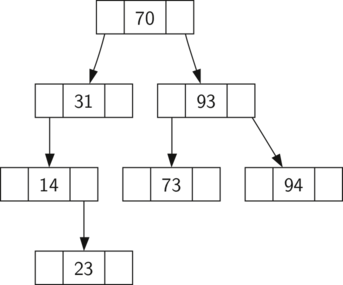Figure 1: A Simple Binary Search Tree

Now that you know what a binary search tree is, we will look at how a binary search tree is constructed. The search tree in Figure 1 represents the nodes that exist after we have inserted the following keys in the order shown: $$70,31,93,94,14,23,73$$. Since 70 was the first key inserted into the tree, it is the root. Next, 31 is less than 70, so it becomes the left child of 70. Next, 93 is greater than 70, so it becomes the right child of 70. Now we have two levels of the tree filled, so the next key is going to be the left or right child of either 31 or 93. Since 94 is greater than 70 and 93, it becomes the right child of 93. Similarly 14 is less than 70 and 31, so it becomes the left child of 31. 23 is also less than 31, so it must be in the left subtree of 31. However, it is greater than 14, so it becomes the right child of 14.

To implement the binary search tree, we will use the nodes and references approach similar to the one we used to implement the linked list, and the expression tree. However, because we must be able to create and work with a binary search tree that is empty, our implementation will use two classes. The first class we will call BinarySearchTree, and the second class we will call TreeNode. The BinarySearchTree class has a reference to the TreeNode that is the root of the binary search tree. In most cases the external methods defined in the outer class simply check to see if the tree is empty. If there are nodes in the tree, the request is just passed on to a private method defined in the BinarySearchTree class that takes the root as a parameter. In the case where the tree is empty or we want to delete the key at the root of the tree, we must take special action. The code for the BinarySearchTree class constructor along with a few other miscellaneous functions is shown in Listing 1.

Q-1: How many children can a node have in a binary search tree?

• At least 4
• Incorrect. Refer back to the definition of a binary search tree.
• At most 3
• Incorrect.
• At least 1
• Incorrect, it has a limit.
• At most 2
• Correct!

Listing 1

class BinarySearchTree{
private:
TreeNode *root;
int size;

public:
BinarySearchTree(){
this->root = NULL;
this->size = 0;
}

int length(){
return this->size;
}
}


The TreeNode class provides many helper functions that make the work done in the BinarySearchTree class methods much easier. The constructor for a TreeNode, along with these helper functions, is shown in Listing 2. As you can see in the listing many of these helper functions help to classify a node according to its own position as a child, (left or right) and the kind of children the node has. The TreeNode class will also explicitly keep track of the parent as an attribute of each node. You will see why this is important when we discuss the implementation for the del operator.

Another interesting aspect of the implementation of TreeNode in Listing 2 is that we use C++’s optional parameters. Optional parameters make it easy for us to create a TreeNode under several different circumstances. Sometimes we will want to construct a new TreeNode that already has both a parent and a child. With an existing parent and child, we can pass parent and child as parameters. At other times we will just create a TreeNode with the key value pair, and we will not pass any parameters for parent or child. In this case, the default values of the optional parameters are used.

Listing 2

class TreeNode{
public:
int key;
TreeNode *leftChild;
TreeNode *rightChild;
TreeNode *parent;

TreeNode(int key, string val, TreeNode *parent = NULL, TreeNode *left = NULL, TreeNode *right = NULL){
this->key = key;
this->leftChild = left;
this->rightChild = right;
this->parent = parent;
}

TreeNode *hasLeftChild(){
return this->leftChild;
}

TreeNode *hasRightChild(){
return this->rightChild;
}

bool isLeftChild(){
return this->parent && this->parent->leftChild == this;
}

bool isRightChild(){
return this->parent && this->parent->rightChild == this;
}

bool isRoot(){
return !this->parent;
}

bool isLeaf(){
return !(this->rightChild || this->leftChild);
}

bool hasAnyChildren(){
return this->rightChild || this->leftChild;
}

bool hasBothChildren(){
return this->rightChild && this->leftChild;
}

void replaceNodeData(int key, string value, TreeNode *lc = NULL, TreeNode *rc = NULL){
this->key = key;
this->leftChild = lc;
this->rightChild = rc;
if (this->hasLeftChild()){
this->leftChild->parent = this;
}
if (this->hasRightChild()){
this->rightChild->parent = this;
}
}
}


Now that we have the BinarySearchTree shell and the TreeNode it is time to write the put method that will allow us to build our binary search tree. The put method is a method of the BinarySearchTree class. This method will check to see if the tree already has a root. If there is not a root then put will create a new TreeNode and install it as the root of the tree. If a root node is already in place then put calls the private, recursive, helper function _put to search the tree according to the following algorithm:

• Starting at the root of the tree, search the binary tree comparing the new key to the key in the current node. If the new key is less than the current node, search the left subtree. If the new key is greater than the current node, search the right subtree.

• When there is no left (or right) child to search, we have found the position in the tree where the new node should be installed.

• To add a node to the tree, create a new TreeNode object and insert the object at the point discovered in the previous step.

Listing 3 shows the C++ code for inserting a new node in the tree. The _put function is written recursively following the steps outlined above. Notice that when a new child is inserted into the tree, the currentNode is passed to the new tree as the parent.

One important problem with our implementation of insert is that duplicate keys are not handled properly. As our tree is implemented a duplicate key will create a new node with the same key value in the right subtree of the node having the original key. The result of this is that the node with the new key will never be found during a search. A better way to handle the insertion of a duplicate key is for the value associated with the new key to replace the old value. We leave fixing this bug as an exercise for you.

Listing 3

void put(int key, string val){
if (this->root){
this->_put(key, val, this->root);
}
else{
this->root = new TreeNode(key, val);
}
this->size = this->size + 1;
}

void _put(int key, string val, TreeNode *currentNode){
if (key < currentNode->key){
if (currentNode->hasLeftChild()){
this->_put(key, val, currentNode->leftChild);
}
else{
currentNode->leftChild = new TreeNode(key, val, currentNode);
}
}
else{
if (currentNode->hasRightChild()){
this->_put(key, val, currentNode->rightChild);
}
else{
currentNode->rightChild = new TreeNode(key, val, currentNode);
}
}
}


Figure 2 illustrates the process for inserting a new node into a binary search tree. The lightly shaded nodes indicate the nodes that were visited during the insertion process.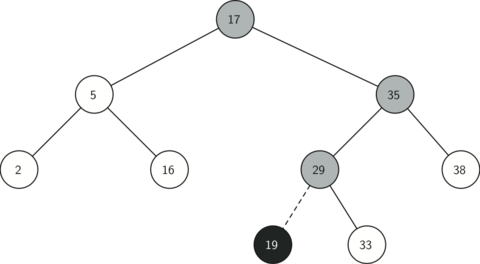Figure 2: Inserting a Node with Key = 19

Self Check

Q-2: Which of the trees shows a correct binary search tree given that the keys were inserted in the following order 5, 30, 2, 40, 25, 4.

•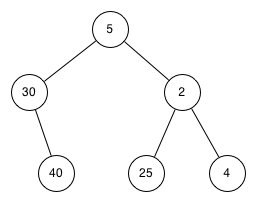• Remember, starting at the root keys less than the root must be in the left subtree, while keys greater than the root go in the right subtree.
•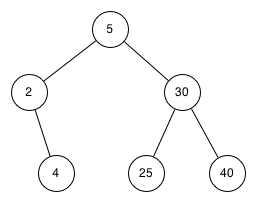• good job.
•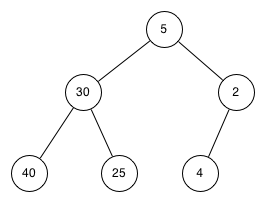• This looks like a binary tree that satisfies the full tree property needed for a heap.

Once the tree is constructed, the next task is to implement the retrieval of a value for a given key. The get method is even easier than the put method because it simply searches the tree recursively until it gets to a non-matching leaf node or finds a matching key. When a matching key is found, the value stored in the payload of the node is returned.

Listing 5 shows the code for get and _get. The search code in the _get method uses the same logic for choosing the left or right child as the _put method. Notice that the _get method returns a TreeNode to get, this allows _get to be used as a flexible helper method for other BinarySearchTree methods that may need to make use of other data from the TreeNode besides the payload.

Listing 5

string get(int key){
if (this->root){
TreeNode *res = this->_get(key, this->root);
if (res){
}
else{
return 0;
}
}
else{
return 0;
}
}

TreeNode  *_get(int key, TreeNode *currentNode){
if (!currentNode){
return NULL;
}
else if (currentNode->key == key){
return currentNode;
}
else if (key < currentNode->key){
return this->_get(key, currentNode->leftChild);
}
else{
return this->_get(key, currentNode->rightChild);
}
}


Finally, we turn our attention to the most challenging method in the binary search tree, the deletion of a key (see Listing 7). The first task is to find the node to delete by searching the tree. If the tree has more than one node we search using the _get method to find the TreeNode that needs to be removed. If the tree only has a single node, that means we are removing the root of the tree, but we still must check to make sure the key of the root matches the key that is to be deleted. In either case if the key is not found the del operator raises an error.

Listing 7

void del(int key){
if (this->size > 1){
TreeNode *nodeToRemove = this->_get(key, this->root);
if (nodeToRemove){
this->remove(nodeToRemove);
this->size = this->size - 1;
}
else{
cerr << "Error, key not in tree" << endl;
}
}
else if (this->size == 1 && this->root->key == key){
this->root = NULL;
this->size = this->size - 1;
}
else{
cerr << "Error, key not in tree" << endl;
}
}


Once we’ve found the node containing the key we want to delete, there are three cases that we must consider:

1. The node to be deleted has no children (see Figure 3).

2. The node to be deleted has only one child (see Figure 4).

3. The node to be deleted has two children (see Figure 5).

The first case is straightforward (see Listing 8). If the current node has no children all we need to do is delete the node and remove the reference to this node in the parent. The code for this case is shown in here.

Listing 8

if (currentNode->isLeaf()){ //leaf
if (currentNode == currentNode->parent->leftChild){
currentNode->parent->leftChild = NULL;
}
else{
currentNode->parent->rightChild = NULL;
}
}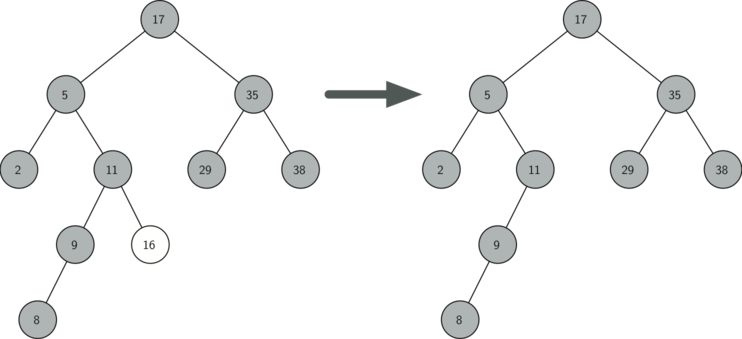Figure 3: Deleting Node 16, a Node without Children

The second case is only slightly more complicated (see Listing 10). If a node has only a single child, then we can simply promote the child to take the place of its parent. The code for this case is shown in the next listing. As you look at this code you will see that there are six cases to consider. Since the cases are symmetric with respect to either having a left or right child we will just discuss the case where the current node has a left child. The decision proceeds as follows:

1. If the current node is a left child then we only need to update the parent reference of the left child to point to the parent of the current node, and then update the left child reference of the parent to point to the current node’s left child.

2. If the current node is a right child then we only need to update the parent reference of the left child to point to the parent of the current node, and then update the right child reference of the parent to point to the current node’s left child.

3. If the current node has no parent, it must be the root. In this case we will just replace the key, payload, leftChild, and rightChild data by calling the replaceNodeData method on the root.

Listing 10

else{ // this node has one child
if (currentNode->hasLeftChild()){
if (currentNode->isLeftChild()){
currentNode->leftChild->parent = currentNode->parent;
currentNode->parent->leftChild = currentNode->leftChild;
}
else if (currentNode->isRightChild()){
currentNode->leftChild->parent = currentNode->parent;
currentNode->parent->rightChild = currentNode->leftChild;
}
else{
currentNode->replaceNodeData(currentNode->leftChild->key,
currentNode->leftChild->leftChild,
currentNode->leftChild->rightChild);

}
}
else{
if (currentNode->isLeftChild()){
currentNode->rightChild->parent = currentNode->parent;
currentNode->parent->leftChild = currentNode->rightChild;
}
else if (currentNode->isRightChild()){
currentNode->rightChild->parent = currentNode->parent;
currentNode->parent->rightChild = currentNode->rightChild;
}
else{
currentNode->replaceNodeData(currentNode->rightChild->key,
currentNode->rightChild->leftChild,
currentNode->rightChild->rightChild);
}
}
}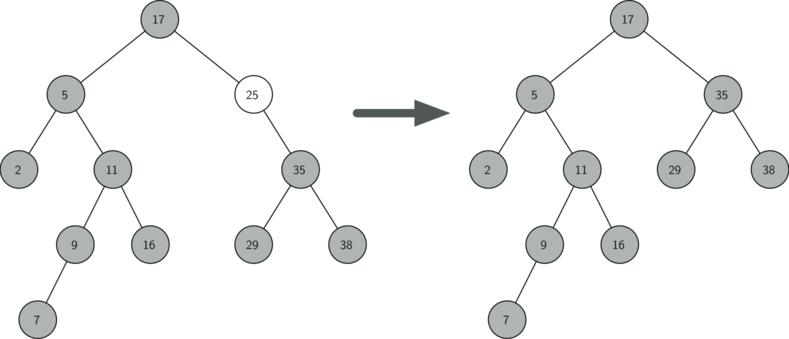Figure 4: Deleting Node 25, a Node That Has a Single Child

The third case is the most difficult case to handle (see Listing 10). If a node has two children, then it is unlikely that we can simply promote one of them to take the node’s place. We can, however, search the tree for a node that can be used to replace the one scheduled for deletion. What we need is a node that will preserve the binary search tree relationships for both of the existing left and right subtrees. The node that will do this is the node that has the next-largest key in the tree. We call this node the successor, and we will look at a way to find the successor shortly. The successor is guaranteed to have no more than one child, so we know how to remove it using the two cases for deletion that we have already implemented. Once the successor has been removed, we simply put it in the tree in place of the node to be deleted.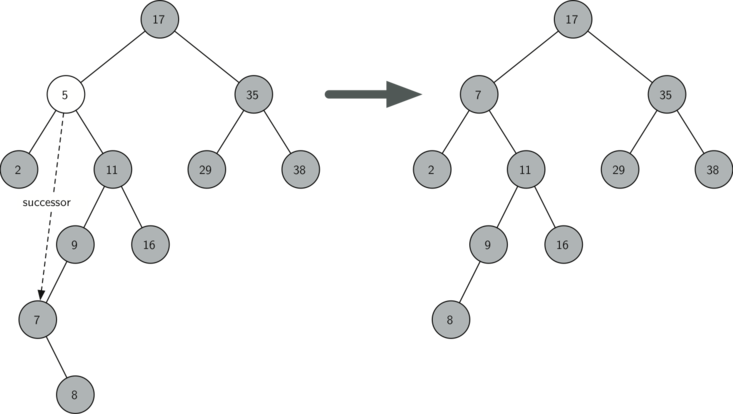Figure 5: Deleting Node 5, a Node with Two Children

The code to handle the third case is shown in the next listing. Notice that we make use of the helper methods findSuccessor and findMin to find the successor. To remove the successor, we make use of the method spliceOut. The reason we use spliceOut is that it goes directly to the node we want to splice out and makes the right changes. We could call delete recursively, but then we would waste time re-searching for the key node.

Listing 11

else if (currentNode->hasBothChildren()){ //interior
TreeNode *succ = currentNode->findSuccessor();
succ->spliceOut();
currentNode->key = succ->key;
}


The code to find the successor is shown below (see Listing 12) and as you can see is a method of the TreeNode class. This code makes use of the same properties of binary search trees that cause an inorder traversal to print out the nodes in the tree from smallest to largest. There are three cases to consider when looking for the successor:

1. If the node has a right child, then the successor is the smallest key in the right subtree.

2. If the node has no right child and is the left child of its parent, then the parent is the successor.

3. If the node is the right child of its parent, and itself has no right child, then the successor to this node is the successor of its parent, excluding this node.

The first condition is the only one that matters for us when deleting a node from a binary search tree. However, the findSuccessor method has other uses that we will explore in the exercises at the end of this chapter.

The findMin method is called to find the minimum key in a subtree. You should convince yourself that the minimum valued key in any binary search tree is the leftmost child of the tree. Therefore the findMin method simply follows the leftChild references in each node of the subtree until it reaches a node that does not have a left child.

Listing 12

TreeNode *findSuccessor(){
TreeNode *succ = NULL;
if (this->hasRightChild()){
succ = this->rightChild->findMin();
}
else{
if (this->parent){
if (this->isLeftChild()){
succ = this->parent;
}
else{
this->parent->rightChild = NULL;
succ = this->parent->findSuccessor();
this->parent->rightChild = this;
}
}
}
return succ;
}

TreeNode *findMin(){
TreeNode *current = this;
while (current->hasLeftChild()){
current = current->leftChild;
}
return current;
}

void spliceOut(){
if (this->isLeaf()){
if (this->isLeftChild()){
this->parent->leftChild = NULL;
}
else{
this->parent->rightChild = NULL;
}
}
else if (this->hasAnyChildren()){
if (this->hasLeftChild()){
if (this->isLeftChild()){
this->parent->leftChild = this->leftChild;
}
else{
this->parent->rightChild = this->rightChild;
}
this->leftChild->parent = this->parent;
}
else{
if (this->isLeftChild()){
this->parent->leftChild = this->rightChild;
}
else{
this->parent->rightChild = this->rightChild;
}
this->rightChild->parent = this->parent;
}
}
}


We need to look at one last interface method for the binary search tree. Suppose that we would like to simply iterate over all the keys in the tree in order. This is definitely something we have done with dictionaries, so why not trees? You already know how to traverse a binary tree in order, using the inorder traversal algorithm. However, writing an iterator requires a bit more work, since an iterator should return only one node each time the iterator is called.

Python provides us with a very powerful function to use when creating an iterator. The function is called yield. yield is similar to return in that it returns a value to the caller. However, yield also takes the additional step of freezing the state of the function so that the next time the function is called it continues executing from the exact point it left off earlier. Functions that create objects that can be iterated are called generator functions.

The code for an inorder iterator of a binary tree is shown in the next listing. Look at this code carefully; at first glance you might think that the code is not recursive. However, remember that __iter__ overrides the for x in operation for iteration, so it really is recursive! Because it is recursive over TreeNode instances the __iter__ method is defined in the TreeNode class.

def __iter__(self):
if self:
if self.hasLeftChild():
for elem in self.leftChiLd:
yield elem
yield self.key
if self.hasRightChild():
for elem in self.rightChild:
yield elem


At this point you may want to download the entire file containing the full version of the BinarySearchTree and TreeNode classes.